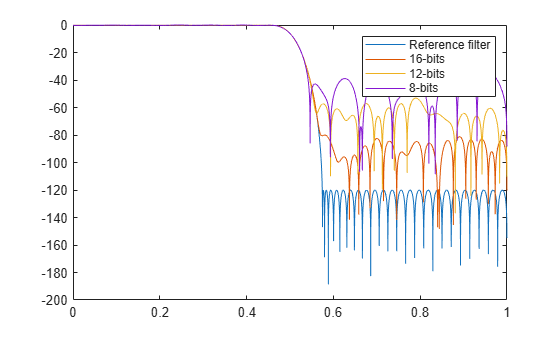Documentation

# reffilter

Reference filter for fixed-point or single-precision filter

## Syntax

```href = reffilter(hd) ```

## Description

`href = reffilter(hd)` returns a new filter `href` that has the same structure as `hd`, but uses the reference coefficients and has its arithmetic property set to `double`. Note that `hd` can be either a fixed-point filter (arithmetic property set to '`fixed`', or a single-precision floating-point filter whose arithmetic property is '`single`').

`reffilter(hd)` differs from `double``(hd)` in that

• the filter `href` returned by `reffilter` has the reference coefficients of `hd`.

• `double(hd)` returns the quantized coefficients of `hd` represented in double-precision.

To check the performance of your fixed-point filter, use `href` = `reffilter(hd)` to quickly have the floating-point, double-precision version of `hd` available for comparison.

## Examples

collapse all

Compare several fixed-point quantizations of a filter with the same double-precision floating-point version of the filter.

```h = dfilt.dffir(firceqrip(87,.5,[1e-3,1e-6])); % Lowpass filter. h1 = copy(h); h2 = copy(h); % Create copies of h. h.arithmetic = 'fixed'; % Set h to filter using fixed-point... % arithmetic. h1.arithmetic = 'fixed'; % Same for h1. h2.arithmetic = 'fixed'; % Same for h2. h.CoeffWordLength = 16; % Use 16 bits to represent the... % coefficients. h1.CoeffWordLength = 12; % Use 12 bits to represent the... % coefficients. h2.CoeffWordLength = 8; % Use 8 bits to represent the... % coefficients. href = reffilter(h); hfvt = fvtool(href,h,h1,h2); set(hfvt,'ShowReference','off'); % Reference displayed once % already. legend(hfvt,'Reference filter','16-bits','12-bits','8-bits');```The fvtool shows `href`, the reference filter, and the effects of using three different word lengths to represent the coefficients.